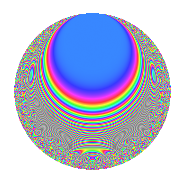# Properties

 Label 1005.2.aLevel 1005 Weight 2 Character orbit a Rep. character $$\chi_{1005}(1,\cdot)$$ Character field $$\Q$$ Dimension 43 Newforms 10 Sturm bound 272 Trace bound 2

# Learn more about

## Defining parameters

 Level: $$N$$ = $$1005 = 3 \cdot 5 \cdot 67$$ Weight: $$k$$ = $$2$$ Character orbit: $$[\chi]$$ = 1005.a (trivial) Character field: $$\Q$$ Newforms: $$10$$ Sturm bound: $$272$$ Trace bound: $$2$$ Distinguishing $$T_p$$: $$2$$

## Dimensions

The following table gives the dimensions of various subspaces of $$M_{2}(\Gamma_0(1005))$$.

Total New Old
Modular forms 140 43 97
Cusp forms 133 43 90
Eisenstein series 7 0 7

The following table gives the dimensions of the cuspidal new subspaces with specified eigenvalues for the Atkin-Lehner operators and the Fricke involution.

$$3$$$$5$$$$67$$FrickeDim.
$$+$$$$+$$$$+$$$$+$$$$4$$
$$+$$$$+$$$$-$$$$-$$$$8$$
$$+$$$$-$$$$+$$$$-$$$$5$$
$$+$$$$-$$$$-$$$$+$$$$5$$
$$-$$$$+$$$$+$$$$-$$$$5$$
$$-$$$$+$$$$-$$$$+$$$$5$$
$$-$$$$-$$$$+$$$$+$$$$4$$
$$-$$$$-$$$$-$$$$-$$$$7$$
Plus space$$+$$$$18$$
Minus space$$-$$$$25$$

## Trace form

 $$43q$$ $$\mathstrut -\mathstrut 3q^{2}$$ $$\mathstrut -\mathstrut q^{3}$$ $$\mathstrut +\mathstrut 41q^{4}$$ $$\mathstrut -\mathstrut q^{5}$$ $$\mathstrut +\mathstrut q^{6}$$ $$\mathstrut -\mathstrut 8q^{7}$$ $$\mathstrut -\mathstrut 15q^{8}$$ $$\mathstrut +\mathstrut 43q^{9}$$ $$\mathstrut +\mathstrut O(q^{10})$$ $$43q$$ $$\mathstrut -\mathstrut 3q^{2}$$ $$\mathstrut -\mathstrut q^{3}$$ $$\mathstrut +\mathstrut 41q^{4}$$ $$\mathstrut -\mathstrut q^{5}$$ $$\mathstrut +\mathstrut q^{6}$$ $$\mathstrut -\mathstrut 8q^{7}$$ $$\mathstrut -\mathstrut 15q^{8}$$ $$\mathstrut +\mathstrut 43q^{9}$$ $$\mathstrut +\mathstrut 5q^{10}$$ $$\mathstrut -\mathstrut 4q^{11}$$ $$\mathstrut -\mathstrut 7q^{12}$$ $$\mathstrut -\mathstrut 14q^{13}$$ $$\mathstrut +\mathstrut 3q^{15}$$ $$\mathstrut +\mathstrut 41q^{16}$$ $$\mathstrut -\mathstrut 14q^{17}$$ $$\mathstrut -\mathstrut 3q^{18}$$ $$\mathstrut +\mathstrut 9q^{20}$$ $$\mathstrut -\mathstrut 8q^{21}$$ $$\mathstrut -\mathstrut 28q^{22}$$ $$\mathstrut -\mathstrut 4q^{23}$$ $$\mathstrut -\mathstrut 3q^{24}$$ $$\mathstrut +\mathstrut 43q^{25}$$ $$\mathstrut +\mathstrut 38q^{26}$$ $$\mathstrut -\mathstrut q^{27}$$ $$\mathstrut -\mathstrut 16q^{28}$$ $$\mathstrut -\mathstrut 26q^{29}$$ $$\mathstrut -\mathstrut 3q^{30}$$ $$\mathstrut +\mathstrut 17q^{32}$$ $$\mathstrut +\mathstrut 4q^{33}$$ $$\mathstrut +\mathstrut 26q^{34}$$ $$\mathstrut +\mathstrut 41q^{36}$$ $$\mathstrut -\mathstrut 2q^{37}$$ $$\mathstrut +\mathstrut 52q^{38}$$ $$\mathstrut -\mathstrut 14q^{39}$$ $$\mathstrut +\mathstrut 9q^{40}$$ $$\mathstrut +\mathstrut 6q^{41}$$ $$\mathstrut -\mathstrut 8q^{42}$$ $$\mathstrut -\mathstrut 28q^{43}$$ $$\mathstrut -\mathstrut 4q^{44}$$ $$\mathstrut -\mathstrut q^{45}$$ $$\mathstrut +\mathstrut 32q^{46}$$ $$\mathstrut -\mathstrut 20q^{47}$$ $$\mathstrut +\mathstrut q^{48}$$ $$\mathstrut +\mathstrut 27q^{49}$$ $$\mathstrut -\mathstrut 3q^{50}$$ $$\mathstrut -\mathstrut 2q^{51}$$ $$\mathstrut -\mathstrut 26q^{52}$$ $$\mathstrut -\mathstrut 30q^{53}$$ $$\mathstrut +\mathstrut q^{54}$$ $$\mathstrut +\mathstrut 4q^{55}$$ $$\mathstrut +\mathstrut 48q^{56}$$ $$\mathstrut -\mathstrut 20q^{57}$$ $$\mathstrut +\mathstrut 30q^{58}$$ $$\mathstrut -\mathstrut 16q^{59}$$ $$\mathstrut +\mathstrut 5q^{60}$$ $$\mathstrut -\mathstrut 30q^{61}$$ $$\mathstrut +\mathstrut 16q^{62}$$ $$\mathstrut -\mathstrut 8q^{63}$$ $$\mathstrut +\mathstrut q^{64}$$ $$\mathstrut +\mathstrut 10q^{65}$$ $$\mathstrut +\mathstrut 20q^{66}$$ $$\mathstrut +\mathstrut 7q^{67}$$ $$\mathstrut -\mathstrut 14q^{68}$$ $$\mathstrut +\mathstrut 8q^{69}$$ $$\mathstrut +\mathstrut 16q^{70}$$ $$\mathstrut +\mathstrut 24q^{71}$$ $$\mathstrut -\mathstrut 15q^{72}$$ $$\mathstrut -\mathstrut 6q^{73}$$ $$\mathstrut -\mathstrut 34q^{74}$$ $$\mathstrut -\mathstrut q^{75}$$ $$\mathstrut +\mathstrut 76q^{76}$$ $$\mathstrut -\mathstrut 16q^{77}$$ $$\mathstrut -\mathstrut 2q^{78}$$ $$\mathstrut -\mathstrut 48q^{79}$$ $$\mathstrut +\mathstrut q^{80}$$ $$\mathstrut +\mathstrut 43q^{81}$$ $$\mathstrut +\mathstrut 66q^{82}$$ $$\mathstrut +\mathstrut 20q^{83}$$ $$\mathstrut +\mathstrut 16q^{84}$$ $$\mathstrut -\mathstrut 2q^{85}$$ $$\mathstrut +\mathstrut 4q^{86}$$ $$\mathstrut -\mathstrut 22q^{87}$$ $$\mathstrut +\mathstrut 4q^{88}$$ $$\mathstrut +\mathstrut 42q^{89}$$ $$\mathstrut +\mathstrut 5q^{90}$$ $$\mathstrut -\mathstrut 8q^{91}$$ $$\mathstrut -\mathstrut 104q^{92}$$ $$\mathstrut -\mathstrut 24q^{93}$$ $$\mathstrut -\mathstrut 40q^{94}$$ $$\mathstrut -\mathstrut 20q^{95}$$ $$\mathstrut +\mathstrut 5q^{96}$$ $$\mathstrut +\mathstrut 14q^{97}$$ $$\mathstrut -\mathstrut 11q^{98}$$ $$\mathstrut -\mathstrut 4q^{99}$$ $$\mathstrut +\mathstrut O(q^{100})$$

## Decomposition of $$S_{2}^{\mathrm{new}}(\Gamma_0(1005))$$ into irreducible Hecke orbits

Label Dim. $$A$$ Field CM Traces A-L signs $q$-expansion
$$a_2$$ $$a_3$$ $$a_5$$ $$a_7$$ 3 5 67
1005.2.a.a $$1$$ $$8.025$$ $$\Q$$ None $$0$$ $$1$$ $$-1$$ $$2$$ $$-$$ $$+$$ $$-$$ $$q+q^{3}-2q^{4}-q^{5}+2q^{7}+q^{9}-6q^{11}+\cdots$$
1005.2.a.b $$1$$ $$8.025$$ $$\Q$$ None $$1$$ $$-1$$ $$1$$ $$0$$ $$+$$ $$-$$ $$+$$ $$q+q^{2}-q^{3}-q^{4}+q^{5}-q^{6}-3q^{8}+\cdots$$
1005.2.a.c $$4$$ $$8.025$$ 4.4.1957.1 None $$-4$$ $$4$$ $$4$$ $$-9$$ $$-$$ $$-$$ $$+$$ $$q+(-1+\beta _{1})q^{2}+q^{3}+(1-\beta _{1}+\beta _{2}+\cdots)q^{4}+\cdots$$
1005.2.a.d $$4$$ $$8.025$$ 4.4.2525.1 None $$-2$$ $$4$$ $$-4$$ $$-1$$ $$-$$ $$+$$ $$-$$ $$q-\beta _{1}q^{2}+q^{3}+(1+\beta _{1}+\beta _{2})q^{4}-q^{5}+\cdots$$
1005.2.a.e $$4$$ $$8.025$$ 4.4.1957.1 None $$0$$ $$-4$$ $$-4$$ $$1$$ $$+$$ $$+$$ $$+$$ $$q+\beta _{1}q^{2}-q^{3}+(\beta _{1}+\beta _{2}+\beta _{3})q^{4}+\cdots$$
1005.2.a.f $$4$$ $$8.025$$ 4.4.9301.1 None $$2$$ $$-4$$ $$4$$ $$11$$ $$+$$ $$-$$ $$+$$ $$q+(1+\beta _{2})q^{2}-q^{3}+(2+\beta _{2}-\beta _{3})q^{4}+\cdots$$
1005.2.a.g $$5$$ $$8.025$$ 5.5.273397.1 None $$-2$$ $$-5$$ $$5$$ $$-9$$ $$+$$ $$-$$ $$-$$ $$q-\beta _{1}q^{2}-q^{3}+(1+\beta _{1}+\beta _{2})q^{4}+q^{5}+\cdots$$
1005.2.a.h $$5$$ $$8.025$$ 5.5.772525.1 None $$1$$ $$5$$ $$-5$$ $$-3$$ $$-$$ $$+$$ $$+$$ $$q+\beta _{1}q^{2}+q^{3}+(1+\beta _{2})q^{4}-q^{5}+\beta _{1}q^{6}+\cdots$$
1005.2.a.i $$7$$ $$8.025$$ $$\mathbb{Q}[x]/(x^{7} - \cdots)$$ None $$4$$ $$7$$ $$7$$ $$3$$ $$-$$ $$-$$ $$-$$ $$q+(1-\beta _{1})q^{2}+q^{3}+(2-\beta _{1}+\beta _{2})q^{4}+\cdots$$
1005.2.a.j $$8$$ $$8.025$$ $$\mathbb{Q}[x]/(x^{8} - \cdots)$$ None $$-3$$ $$-8$$ $$-8$$ $$-3$$ $$+$$ $$+$$ $$-$$ $$q-\beta _{1}q^{2}-q^{3}+(1+\beta _{2})q^{4}-q^{5}+\beta _{1}q^{6}+\cdots$$

## Decomposition of $$S_{2}^{\mathrm{old}}(\Gamma_0(1005))$$ into lower level spaces

$$S_{2}^{\mathrm{old}}(\Gamma_0(1005)) \cong$$ $$S_{2}^{\mathrm{new}}(\Gamma_0(15))$$$$^{\oplus 2}$$$$\oplus$$$$S_{2}^{\mathrm{new}}(\Gamma_0(67))$$$$^{\oplus 4}$$$$\oplus$$$$S_{2}^{\mathrm{new}}(\Gamma_0(201))$$$$^{\oplus 2}$$$$\oplus$$$$S_{2}^{\mathrm{new}}(\Gamma_0(335))$$$$^{\oplus 2}$$